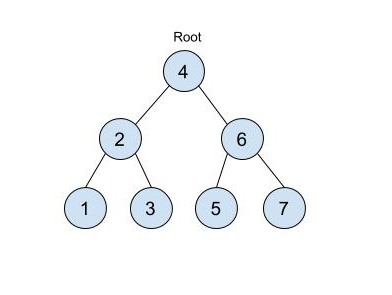# BST Iterator 2

Posted: 1 Apr, 2021
Difficulty: Moderate

## PROBLEM STATEMENT

#### You are given a class named as BSTIterator that represents an iterator over inorder traversal of a binary search tree. You need to implement the following things as follows:

``````1. BSTIterator(Node root) - It is a parameterized constructor in which you are given the root of the Binary search tree. It will be called whenever an object of BSTIterator is created.

2. next() - This member function will return the next element in the in-order traversal of the binary search tree. You need to implement this function.

3. hasNext() - This function will return true if there exists the next element in the traversal else it will return false. You need to implement this function

4. prev() - This member function will return the previous element in the in-order traversal of the binary search tree. You need to implement this function.

5. hasPrev() - This function will return true if there exists the previous element in the traversal else it will return false. You need to implement this function
``````

#### Input Format:

``````The first line of the input contains an integer ‘T’ denoting the number of test cases.

The first line of each test case contains elements of the tree in the level order form. The line consists of values of nodes separated by a single space. In case a node is null, we take -1 in its place.

For example, the input for the tree depicted in the below image would be :
````````````4
2 6
1 3 5 7
-1 -1 -1 -1 -1 -1
``````

#### Explanation :

``````Level 1 :
The root node of the tree is 4

Level 2 :
Left child of 4 = 2
Right child of 4 = 6

Level 3 :
Left child of 2 = 1
Right child of 2 = 3
Left child of 6 = 5
Right child of 6 = 7

Level 4 :
Left child of 1 = null (-1)
Right child of 1 = null (-1)
Left child of 3 = null (-1)
Right child of 3 = null (-1)
Left child of 5 = null (-1)
Right child of 5 = null (-1)
Left child of 7 = null (-1)
Right child of 7 = null (-1)

The first not-null node(of the previous level) is treated as the parent of the first two nodes of the current level. The second not-null node (of the previous level) is treated as the parent node for the next two nodes of the current level and so on.
The input ends when all nodes at the last level are null(-1).
``````
##### Note :
``````The above format was just to provide clarity on how the input is formed for a given tree.

The sequence will be put together in a single line separated by a single space. Hence, for the above-depicted tree, the input will be given as:

4 2 6 1 3 5 7 -1 -1 -1 -1 -1 -1
``````
##### Output Format:
``````For each test case print a single line containing space-separated integers denoting the inorder traversal of the binary search tree.

The output of each test case will be printed in a separate line.
``````
##### Note:
``````You do not need to print anything, it has already been taken care of. Just implement the given function.
``````
##### Constraints:
``````1 <= T <= 10
1 <= N <= 10 ^ 4
1 <= A[i] <= 10 ^ 9

Where ‘T’ is the number of test cases, ‘N’ is the number of nodes, and A[i] is the value of a node.

Time Limit: 1 sec.
``````Approach 1
• We need to implement four functions next(), hasNext(), prev() and hasPrev(). The next() function will move the iterator to the right and return the next element, the hasNext() function will return true if there exists the next element else false, the prev() function will move the iterator to the left and return the previous element, the hasPrev() function will return true if there exists the previous element else false.
• We can prepare a vector that stores all the elements of the binary search tree. We can do so by performing inorder traversal on the given binary search tree and store the elements in the list.
• We maintain a variable to iterate over the list that we prepared in the previous step.
• Now for each call of the next() function, we simply move on the list one step towards the right and return the value stored therein the array.
• For each call of the prev() function, we simply move on the list one step towards the left and return the value stored therein the array.
• For the call of the hasNext() function, we need to check if our iterator reached the end of the array or not. If it is reached to the end of the array that means no element is left we iterate over all the elements so we return false else we will return true.
• Similarly, for the call of the hasPrev() function, we need to check if our iterator is at the start of the array or not. If it is at the start of the array that means no element is present before that element so we return false else we will return true.